# How to Deskew Scanned Documents

Scanned documents often contain skewed or crooked images. They do not look good and are not friendly for OCR.

In this article, we are going to use OpenCV and Python to deskew scanned documents based on text lines.

## Steps to Deskew a Scanned Document Image with OpenCV

We are going to write a Python script to deskew the following sample image.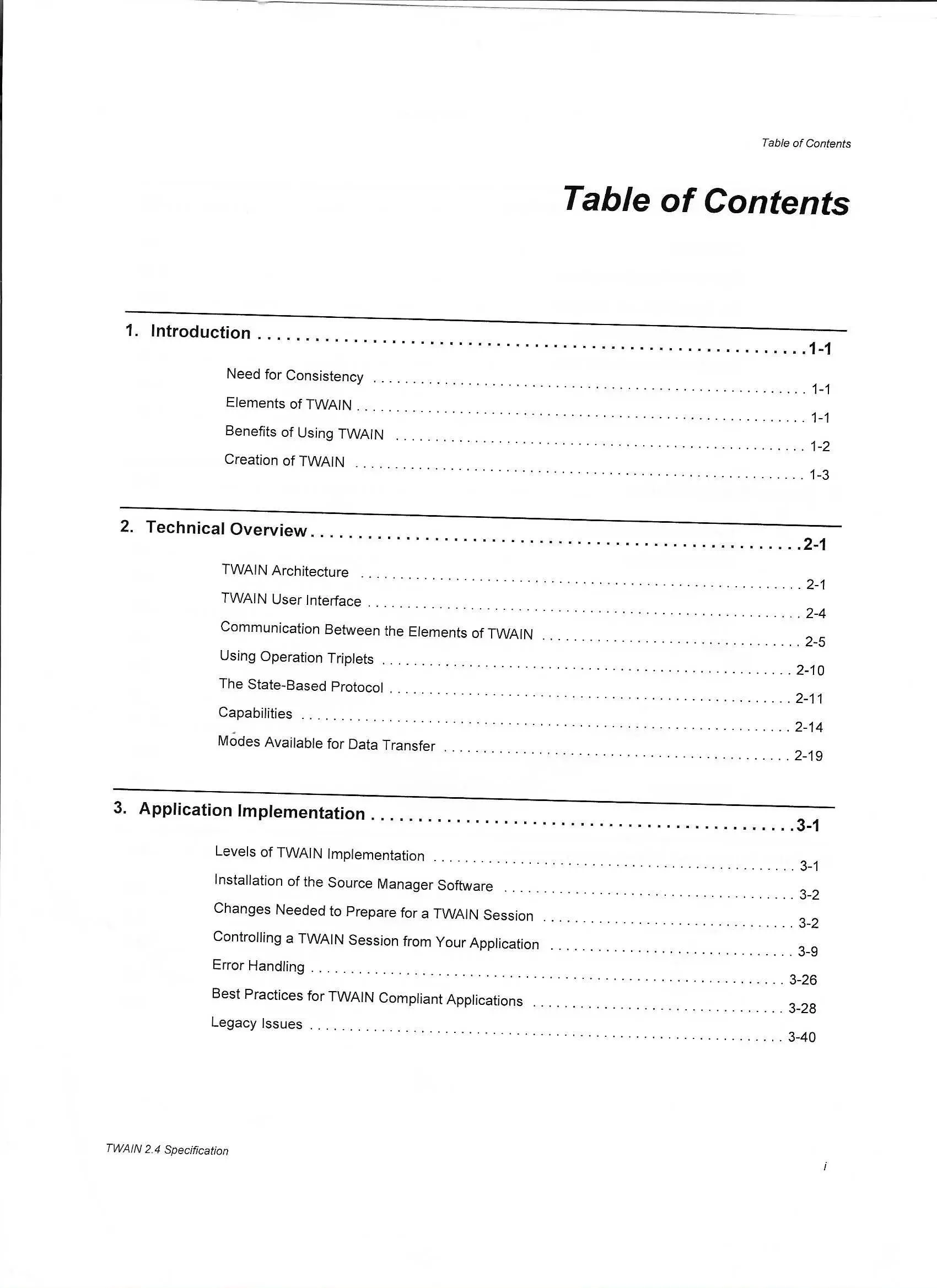### Normalize the Image

1. Scanned images are sharp. We can convert the image to grayscale and blur the image first.

``````img = cv2.imread(path)
gray = cv2.cvtColor(img, cv2.COLOR_BGR2GRAY)
gray = cv2.GaussianBlur(gray, (9, 9), 0)
``````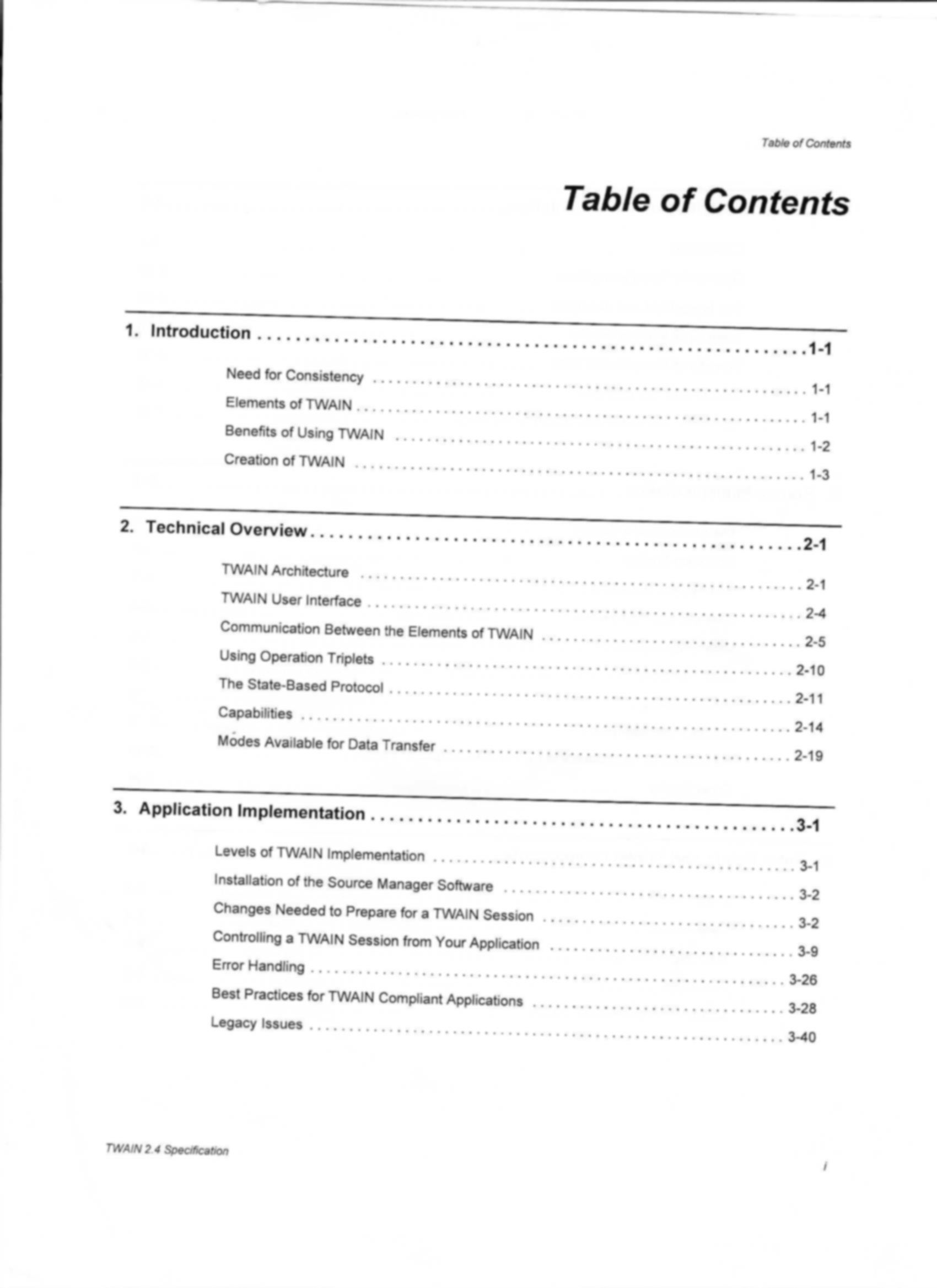2. Resize the image with a fixed height.

``````resized_height = 480
percent = resized_height / len(img)
resized_width = int(percent * len(img))
gray = cv2.resize(gray,(resized_width,resized_height))
``````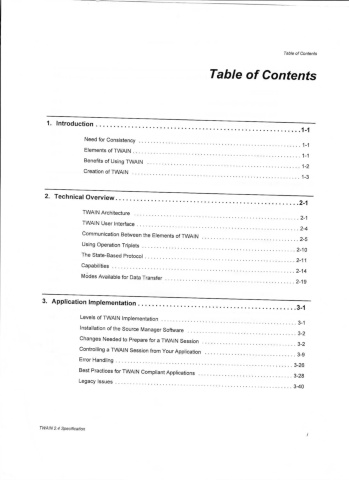3. Draw a rectangle around the border to remove border lines.

``````start_point = (0, 0)
end_point = (gray.shape, gray.shape)
color = (255, 255, 255)
thickness = 10
gray = cv2.rectangle(gray, start_point, end_point, color, thickness)
``````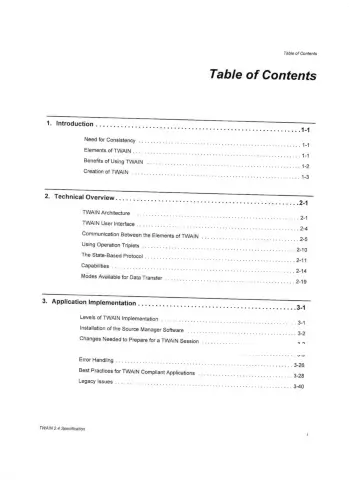4. Invert the image, since we have to process the text.

``````gray = cv2.bitwise_not(gray)
``````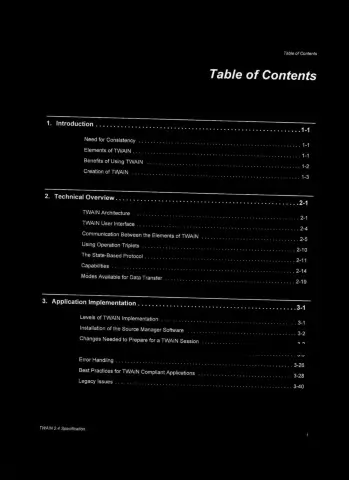5. Run thresholding to get a binary image.

``````thresh = cv2.threshold(gray, 0, 255,
cv2.THRESH_BINARY | cv2.THRESH_OTSU)
``````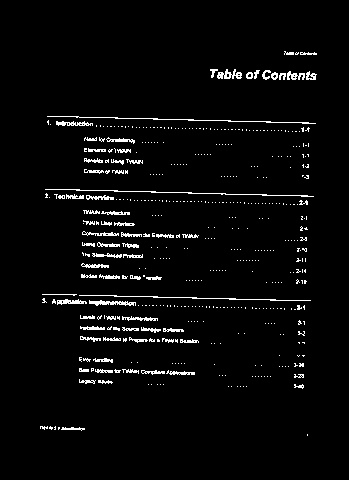6. Dilate the text to make the text lines more obvious.

``````kernel = cv2.getStructuringElement(cv2.MORPH_RECT, (30, 5))
dilate = cv2.dilate(thresh, kernel)
``````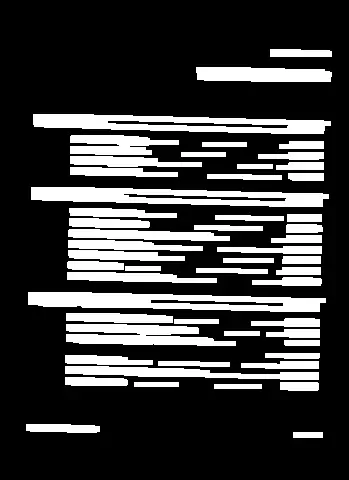### Get the Skewed Angle

1. Find all the contours based on the dilated image.

``````contours, hierarchy = cv2.findContours(dilate, cv2.RETR_LIST, cv2.CHAIN_APPROX_SIMPLE)
``````
2. Use `minAreaRect` to get the rotation angles of contours.

``````angles = []
for contour in contours:
minAreaRect = cv2.minAreaRect(contour)
angle = minAreaRect[-1]
if angle != 90.0 and angle != -0.0: #filter out 0 and 90
angles.append(angle)
``````
3. Use the median as the skewed angle.

``````angles.sort()
mid_angle = angles[int(len(angles)/2)]
``````

### Rotate the Image to Get a Deskewed Image

After getting the skewed angle, we can perform affine transformation to get the deskewed image.

``````if angle > 45: #anti-clockwise
angle = -(90 - angle)
height = original.shape
width = original.shape
m = cv2.getRotationMatrix2D((width / 2, height / 2), angle, 1)
deskewed = cv2.warpAffine(original, m, (width, height), borderValue=(255,255,255))
``````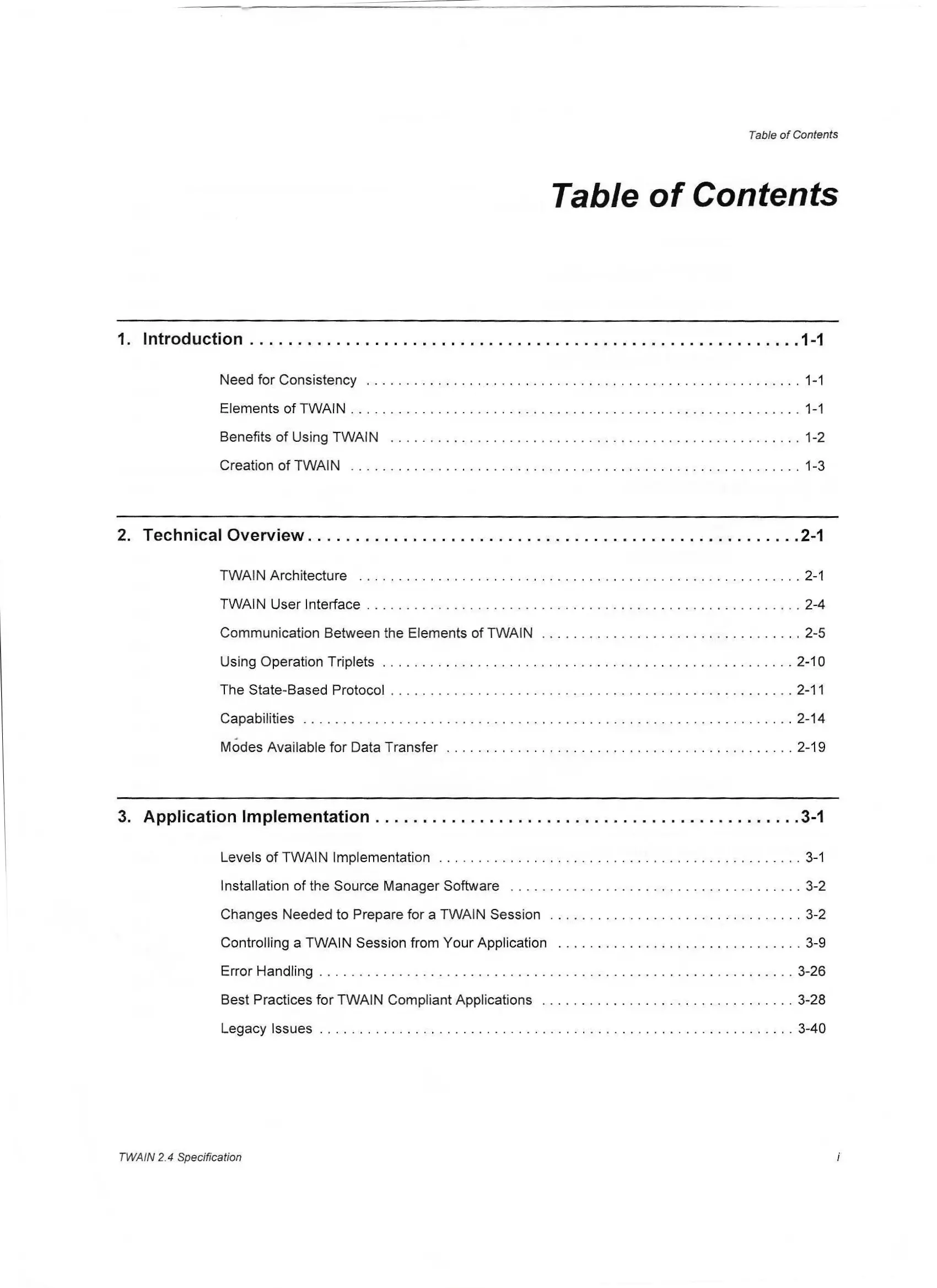## Deskew Scanned Documents using Dynamic Web TWAIN

There are other tools which have the ability to deskew document images. Dynamic Web TWAIN is a JavaScript library to enable document scanning in the browser. It can scan documents from physical scanners via protocols like TWAIN, WIA, SANE and ICA and has a deskew function built-in.

The following is the code snippet to perform deskewing of a scanned document image.

``````function Deskew(index) {
return new Promise((resolve, reject) => {
DWObject.GetSkewAngle(
index,
function(angle) {
console.log("skew angle: " + angle);
DWObject.Rotate(index, angle, true,
function() {
console.log("Successfully deskewed an image!");
resolve();
},
function(errorCode, errorString) {
console.log(errorString);
reject(errorString);
}
);
},
function(errorCode, errorString) {
console.log(errorString);
reject(errorString);
}
);
})
}
``````

You can use this online demo to have a try. It can also load image or PDF files and save documents in a PDF file.

## Source Code

You can find all the code in the following repo:

https://github.com/tony-xlh/deskew